# Common Core: High School - Geometry : Congruence

## Example Questions

### Example Question #11 : Congruence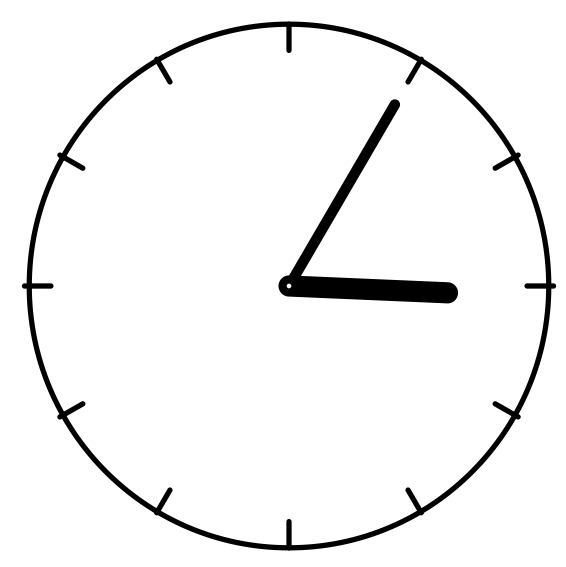Looking at the given clock where the radius isinches, which of the following statements accurately describes the space between the minute and hour hand?

The angle between the minute and hour hand is less thandegrees.

The angle between the hour and minute hand is less thandegrees.

None of the answers.

The angle between the minute and hour hand is less thandegrees.

The angle between the hour and minute hand is less thandegrees.

The angle between the minute and hour hand is less thandegrees.

Explanation:

This question is trying to put a mathematical definition to a real life situation. First, recall the definitions of circles and corresponding angles.

A central angle is known as the angle of a circle where the vertex of the angle is located at the center of the circle.

A circle is composed of 360 degrees.

Also recall that a straight line measures 180 degrees.

Looking at the given clock, it is seen that a straight line can be created by connecting the 12 and 6 on the clock. Since the clock reads 3:05 the angle between the hour and minute hand is less than 180 degrees. Further more, it is less than 45 degrees because the hour hand and minute hand are both between one quadrant of the circle.### Example Question #12 : High School: Geometry

A circular pizza is cut intoequal slices. Which of the following is an accurate mathematical description of one of the pizza slices?

The central angle of one pizza slice isdegrees.

The central angle of one pizza slice isdegrees.

The central angle of one pizza slice is 90 degrees.

None of the answers

The central angle of one pizza slice isdegrees.

The central angle of one pizza slice isdegrees.

Explanation:

This question is trying to put a mathematical definition to a real life situation. First, recall the definitions of circles and corresponding angles.

A central angle is known as the angle of a circle where the vertex of the angle is located at the center of the circle.

A circle is composed of 360 degrees.

Knowing these characteristics, solve for the central angle of one slice of pizza.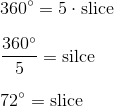Therefore, the correct answer is

"The central angle of one pizza slice is 72 degrees."

### Example Question #1 : Represent And Compare Transformations In The Plane: Ccss.Math.Content.Hsg Co.A.2

A basketball player is able to perfectly spin a basketball on his index finger. While the basketball is spinning, what mathematical expression can be concluded about it?

None of the other answer choices are correct.

The basketball is not a transformation.

The basketball is in rotation.

The basketball is in translation.

The basketball is in reflection.

The basketball is in rotation.

Explanation:

This question is applying mathematical and more specifically, geometric definitions to describe a real life situation. Geometric definitions usually correspond to objects in the 2 dimensional plane or 3 dimensional surface. Since the basket ball is the object in question, this problem is dealing with a the 3 dimensional space.

From here, recall what is given

"A basketball player is able to perfectly spin a basketball on his index finger."

The index finger in this particular situation is acting as an axis for the basketball. Since the basketball is spinning and is maintaining its shape and size, this is considered a "rotation" in geometry.

Therefore, the correct answer is

"The basketball is in rotation"

### Example Question #1 : Represent And Compare Transformations In The Plane: Ccss.Math.Content.Hsg Co.A.2

A trolley car is attached to metal ground rails that go from the most southern block in a city to the most northern block of that same city. If the trolley starts at the south end and moves all the way to the northern end, what type of geometric expression does this situation define?

Translation

Both translation and transformation.

Rotation

Transformation

Reflection

Both translation and transformation.

Explanation:

To answer this question first recall the different geometric definitions that correspond to the listed possible answer choices.

Translation: To move an object from its original position a certain distance without changing the object in any other way.

Transformation: Refers to any of the four changes that can be done to an object geographically. Transformations include, reflections, translations, rotations, and resizing the object.

Reflection: To flip the orientation of an object over a specific line or function.

Rotation: To rotate an object either clockwise or counter clockwise around a center point.

Looking at the given situation,

"A trolley car is attached to metal ground rails that go from the most southern block in a city to the most northern block of that same city. If the trolley starts at the south end and moves all the way to the northern end, what type of geometric expression does this situation define?"

it can be seen that the trolley is not changing shape, it is not rotating nor reflecting. It is however, moving a certain distance from its starting position thus the definition of a translation and a transformation.

### Example Question #1 : Represent And Compare Transformations In The Plane: Ccss.Math.Content.Hsg Co.A.2

Dilation is a type of __________.

None of the answers.

Transformation

Translation

Reflection

Rotation

Transformation

Explanation:

To answer this question first recall the different geometric definitions.

Translation: To move an object from its original position a certain distance without changing the object in any other way.

Transformation: Refers to any of the four changes that can be done to an object geographically. Transformations include, reflections, translations, rotations, and resizing the object.

Reflection: To flip the orientation of an object over a specific line or function.

Rotation: To rotate an object either clockwise or counter clockwise around a center point.

Since dilation is when an object changes size, that means dilation is a type of transformation.

### Example Question #1 : Represent And Compare Transformations In The Plane: Ccss.Math.Content.Hsg Co.A.2

State the transformation of the red rectangle to the black rectangle.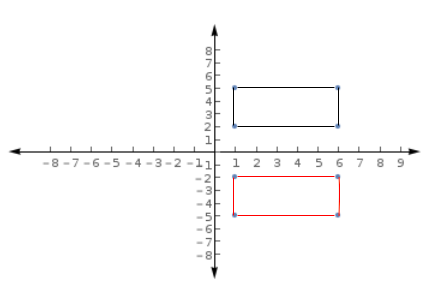Rotation

Translation and Rotation

Translation

Reflection and Translation

None of the other answers

Reflection and Translation

Explanation:

To answer this question first recall the different geometric definitions that correspond to the listed possible answer choices.

Translation: To move an object from its original position a certain distance without changing the object in any other way.

Transformation: Refers to any of the four changes that can be done to an object geographically. Transformations include, reflections, translations, rotations, and resizing the object.

Reflection: To flip the orientation of an object over a specific line or function.

Rotation: To rotate an object either clockwise or counter clockwise around a center point.

Now, examine the given image and choose the appropriate answer.Looking at the red rectangle it is seen that the coordinate points of the vertices are: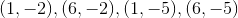The coordinate points of the vertices for the black triangle are: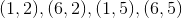Therefore, the red rectangle is being reflected over theaxis or it is being slide up four units. Thus, the correct answer is both a reflection and a translation.

### Example Question #12 : Congruence

Which of the following describes the change in the red polygon to the black polygon?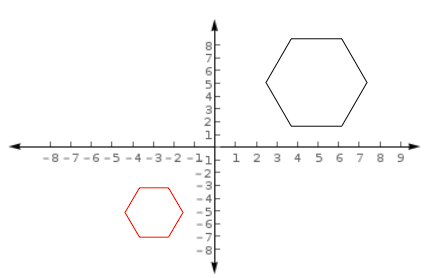Reflection

Reflection and Translation

Rotation

Transformation and Translation

Translation and Rotation

Transformation and Translation

Explanation:

To answer this question first recall the different geometric definitions that correspond to the listed possible answer choices.

Translation: To move an object from its original position a certain distance without changing the object in any other way.

Transformation: Refers to any of the four changes that can be done to an object geographically. Transformations include, reflections, translations, rotations, and resizing the object.

Reflection: To flip the orientation of an object over a specific line or function.

Rotation: To rotate an object either clockwise or counter clockwise around a center point.

Now, examine the given image and choose the appropriate answer.First, it is clear to see that the black polygon is larger than the red polygon. Therefore, a transformation has occurred since the original image has been resized. Also, the black polygon is in quadrant one while the red polygon is in quadrant three, therefore a slide or translation has occurred.

Thus the correct answer is,

"Transformation and Translation"

### Example Question #3 : Represent And Compare Transformations In The Plane: Ccss.Math.Content.Hsg Co.A.2

Which of the following describes the change in the red circle to the black circle?

Assume the center of the circles are the same.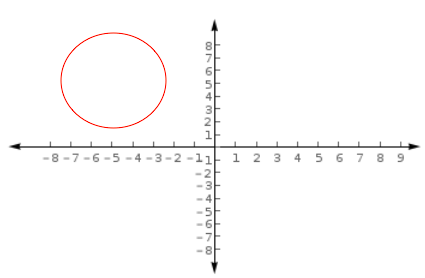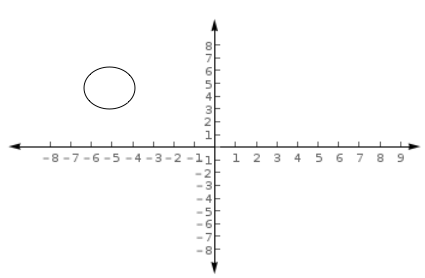Transformation

Rotation

Translation

Translation and Rotation

Reflection

Transformation

Explanation:

To answer this question first recall the different geometric definitions that correspond to the listed possible answer choices.

Translation: To move an object from its original position a certain distance without changing the object in any other way.

Transformation: Refers to any of the four changes that can be done to an object geographically. Transformations include, reflections, translations, rotations, and resizing the object.

Reflection: To flip the orientation of an object over a specific line or function.

Rotation: To rotate an object either clockwise or counter clockwise around a center point.

Now, examine the given image and choose the appropriate answer.First, it is clear to see that the black circle is smaller than the red circle. Therefore, a transformation has occurred since the original image has been resized. Since it is stated that the centers of the circles are the same it can be concluded that only a resizing has occurred.

Thus the correct answer is,

"Transformation"

### Example Question #12 : Congruence

Determine whether the statement is true or false:

When a person's palm is faced down on a desk and that person then flips their hand over, their palm can be described as a reflection.

False

True

False

Explanation:

To answer this question first recall the different geometric definitions that correspond to the listed possible answer choices.

Translation: To move an object from its original position a certain distance without changing the object in any other way.

Transformation: Refers to any of the four changes that can be done to an object geographically. Transformations include, reflections, translations, rotations, and resizing the object.

Reflection: To flip the orientation of an object over a specific line or function.

Rotation: To rotate an object either clockwise or counter clockwise around a center point.

Now look at the specific event in this problem.

"When a person's palm is faced down on a desk and that person then flips their hand over, their palm can be described as a reflection."

Even though reflection is known as the "flipping" of an object it does not describe this situation because the palm of someones hand has different characteristics than the top of the hand therefore, the statement in this particular problem in false.

### Example Question #1 : Represent And Compare Transformations In The Plane: Ccss.Math.Content.Hsg Co.A.2

Which one of the following best describes a rotation?

Slide

Turn

Flip

None of the other answers.

Extend

Turn

Explanation:

To answer this question first recall the different geometric definitions.

Translation: To move an object from its original position a certain distance without changing the object in any other way.

Transformation: Refers to any of the four changes that can be done to an object geographically. Transformations include, reflections, translations, rotations, and resizing the object.

Reflection: To flip the orientation of an object over a specific line or function.

Rotation: To rotate or turn an object either clockwise or counter clockwise around a center point.

Therefore, the English term that best represents rotation is "turn".

### All Common Core: High School - Geometry Resources